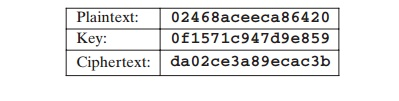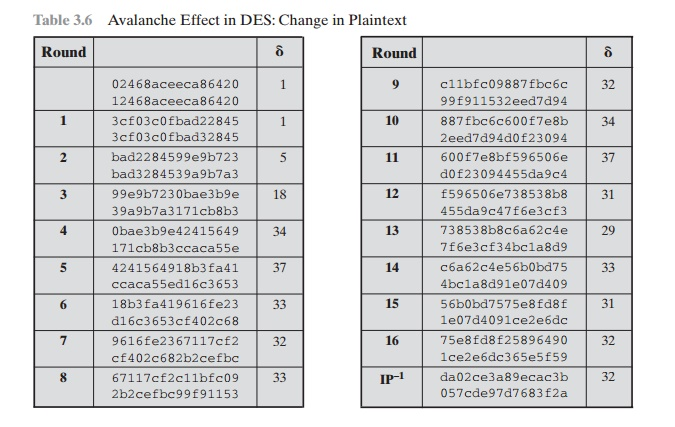Home | | Cryptography and Network Security | A DES(Data Encryption Standard) Example

# A DES(Data Encryption Standard) Example

We now work through an example and consider some of its implications. Although you are not expected to duplicate the example by hand, you will find it informative to study the hex patterns that occur from one step to the next.

A DES EXAMPLE

We now work through an example and consider some of its implications. Although you are not expected to duplicate the example by hand, you will find it informative   to study the hex patterns that occur from one step to the next.

For this example, the plaintext is a hexadecimal palindrome. The plaintext, key, and resulting ciphertext are as follows:Results

Table 3.5 shows the progression of the algorithm. The first row shows the 32-bit values of the left and right halves of data after the initial permutation. The next  16 rows show the results after each round. Also shown is the value of the 48-bit subkey generated for each round. Note that Li = Ri - 1. The final row shows the left- and right-hand values after the inverse initial permutation. These two values com- bined form the ciphertext.

The Avalanche Effect

A desirable property of any encryption algorithm is that a small change in either the plaintext or the key should produce a significant change in the ciphertext. In partic- ular, a change in one bit of the plaintext or one bit of the key should produce a change in many bits of the ciphertext. This is referred to as the avalanche effect. If the change were small, this might provide a way to reduce the size of the plaintext or key space to be searched.

Using the example from Table 3.5, Table 3.6 shows the result when the fourth bit of the plaintext is changed, so that the plaintext is 12468aceeca86420. The second column of the table shows the intermediate 64-bit values at the end of each round for the two plaintexts. The third column shows the number of bits that differ between the two intermediate values. The table shows that, after just three rounds, 18 bits differ between the two blocks. On completion, the two ciphertexts differ in 32 bit positions.

Table 3.5    DES ExampleTable 3.7 shows a similar test using the original plaintext of with two keys that differ in only the fourth bit position: the original key, 0f1571c947d9e859, and the altered key, 1f1571c947d9e859. Again, the results show that about half of the bits in the ciphertext differ and that the avalanche effect is pronounced after just a few rounds.

Table 3.7  Avalanche Effect in DES: Change in KeyStudy Material, Lecturing Notes, Assignment, Reference, Wiki description explanation, brief detail
Cryptography and Network Security Principles and Practice : One Symmetric Ciphers : Block Ciphers and the Data Encryption Standard : A DES(Data Encryption Standard) Example |

Related Topics